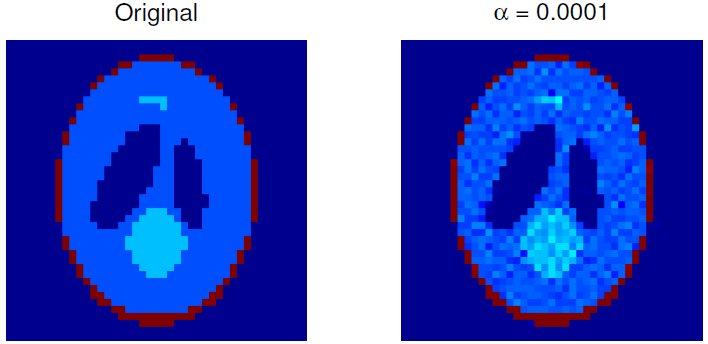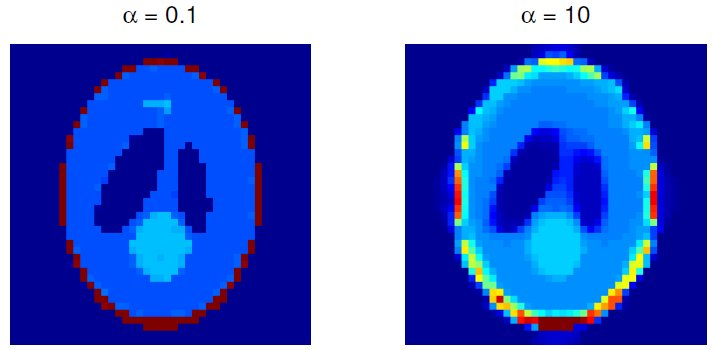3D Total Variation Regularization## TVReg, Version 1.1: Software for 3D Total Variation Regularization

(for Matlab Version 7.5 or later)

TVReg is a software package for 3D tomography using Total Variation regularization. The work was carried out as part of the project CSI: Computational Science in Imaging, which was funded by the Danish Research Council for Technology and Production Sciences, and headed by Prof. Per Christian Hansen, DTU Informatics. The collaborators were DTU Compute, Dept. of Electronic Systems at Aalborg University, and MOSEK ApS.

The software solves the computational problem: minx ½|| A x - b ||22 + α TV(x), where x represents the reconstructed image, b the data, and A the forward operator. Moreover, TV(x) is the Total Variation of the image, and α is a regularization parameter set by the user. The forward operator A can be represented either as a sparse matrix, a point-spread function for convolution-type problems including deblurring, or (from Version 1.1) as a function which applies either the forward operator or its adjoint without necessarily explicitly forming the operator. The latter is useful for large-scale problems where storing the matrix is infeasible.

The main algorithm (UPN) is our practical implementation of an optimal first-order method for strongly convex functions, due to Nesterov, tailored to large-scale total variation regularization. Nesterov's algorithm requires knowledge of both the Lipschitz constant and the strong convexity parameter, both of which are usuall unknown, and our implementation incorporates mechanisms to estimate these important parameters during the iterations - thus making the algorithm suited for practical use. The package also includes two other first-order methods: a method by Nesterov, Beck, and Teboulle (UPN_0) for the case of a zero strong convexity parameter, and the Barzilai-Borwein accelerated gradient projedction method (GPBB).

This is Version 1.1. The core computational routines are written in C, with a mex interface to Matlab.

The algorithm is described in the paper:
• T. L. Jensen, J. H. Jĝrgensen, P. C. Hansen, and S. H. Jensen, Implementation of an Optimal First-Order Method for Strongly Convex Total Variation Regularization, BIT Numerical Mathematics, 52 (2012), pp. 329-356. doi:10.1007/s10543-011-0359-8.
If you publish research based on the use of TVReg please cite this paper.